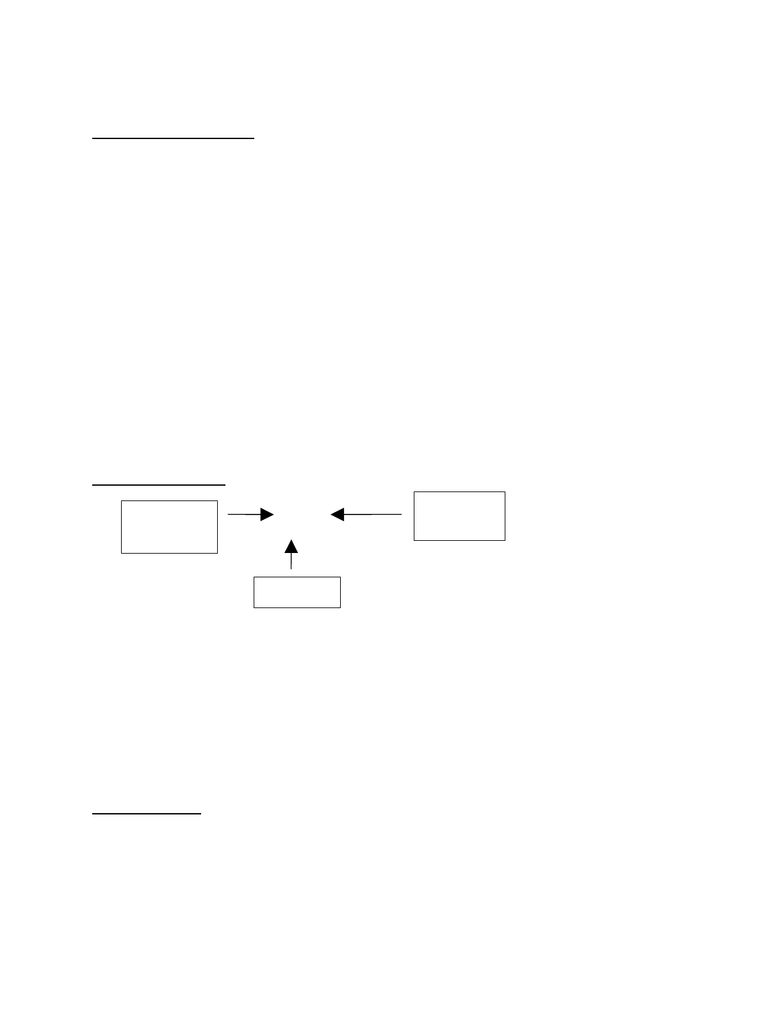Textbook Notes (270,000)
CA (160,000)
UTM (8,000)
Chemistry (100)
d (4)
Chapter 2

CHM110H5 Chapter Notes - Chapter 2: Magnesium Oxide, Antoine Lavoisier, Joseph Proust

Department
Chemistry
Course Code
CHM110H5
Professor
d
Chapter
2

This preview shows page 1. to view the full 5 pages of the document.Chemistry Chapter Two:
Law of Conservation of Mass
In 1774, Antoine Lavoisier (1774-1794) performed an experiment in which he
heated a sealed glass vessel containing a sample of tin and some air. He found that
the glass before heating and the glass after heating were the same. Through further
investigation, he proved that the product of the reaction, tin calx, consisted of the
original tin together with a portion of the air. This proved that oxygen from air is
essential to combustion and it led to the formulation of the Law of Conservation of
Mass: the total mass of substance present after a chemical reaction is the same as
the total mass of substances before the reaction.
Problem: A 0.455g sample of Mg is allowed to burn in 2.315 g of O2 gas. The sole
produce is MgO. After the reaction, no magnesium remains and the mass of
unreacted oxygen is 2.015g. What mass of magnesium oxide is produced?
Solution
Mass before reaction: 0.455g magnesium + 2.315g oxygen = 2.770 g
2.770g mass after reaction =? g magnesium oxide after reaction + 2.015g oxygen
after reaction
? g magnesium oxide after reaction = 2.770 g mass after reaction – 2.015 g oxygen
after reaction = 0.755 g magnesium oxide after reaction.
Law of Constant Composition
In 1799, Joseph Proust: Law of constant composition or law of definite
proportions: All samples of a compound have the same composition – the same
proportions by mass of the constituent elements.
Consider H2O at 10.000g: 1.119 g H = 11.19 % Consider H2O at 27.000g: 3.021
g H = 11.19% 8.881 g O = 88.81%
23.979 g O= 88.81%
Problem: 0.455g of Mg reacted with an excess of oxygen to produce 0.755g of
magnesium oxide. According to the law of constant composition, the mast ratio:
0.455 g magnesium/0.755 g magnesium oxide should exist in all samples of
magnesium oxide. Thus, in 0.500 g of magnesium oxide, the mass of magnesium is:
Solution:
? g magnesium = 0.500 g magnesium oxide x (0.455 g magnesium/0.755 g
magnesium oxide) = 0.301 g magnesium

Unlock to view full version

Only page 1 are available for preview. Some parts have been intentionally blurred.Dalton’s Atomic Theory
1. Each chemical element is composed of minute, indivisible particles
called atoms. Atoms can be neither created nor destroyed during
chemical change.
2. All atoms of an element are alike in mass (weight) and other properties,
but the atoms of one element are different from those of all other
elements.
3. In each of their compounds, different elements combine in a simple
numerical ratio: for example, one atom of A to one of B (AB), or one
atom of A to two of B (AB2).
Daltons 1st assumption explained the law of conservation of mass and his 2nd and 3rd
assumption explained the law of constant composition. This theory led to the
prediction: the law of multiple proportions. If two elements form more than a
single compound, the masses of one element combined with the fixed ratio of the
second are in the ration of small whole numbers (e.g. CO2, N2O, H2O, NO3-)
Chemical Elements
AZE±?
A= mass number and Z= atomic number ***Z is often not specified when writing***
Example: An element with two stable isotopes has 35 protons. The separate isotopes
contain 44 and 46 neutrons. What is the element and write the symbol for the two
isotopes.
Solution: 35 protons = 35 electrons. Number 35 on the periodic table is Bromine (Br).
7935E8135E
Isotopic Masses
Problem: Chlorine has two naturally occurring isotopes: Cl-35 with a natural
abundance of 75.77% and an isotopic mass of 34.969 amu and Cl-37 with a natural
abundance of 24.23% and an isotopic mass of 36.966 amu. What is the atomic mass
of chlorine?
# of
protons
# of
protons +
# of
# of
protons – #
of electrons